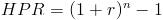# Holding Period ReturnThe formula for the holding period return is used for calculating the return on an investment over multiple periods.

The returns on an investment may be shown on an annual, quarterly, or monthly basis. An individual may be tempted to incorrectly add the percentages of return to find the return over the multiple periods. By incorrectly adding the periodic returns, the effect of compounding is not taken into consideration. For example, a return of 10% the first year followed by a return of 10% the next year does not equate to an aggregate of 20%. By using the holding period return formula, the amount gained would be 21%. The extra 1% can be attributed to the effect of compounding through earning 10% in the second year on the 10% that was earned in the first year. This is sometimes called earning interest on interest.

## Example of Holding Period Return Formula

An example of the holding period return formula would be an investment in an asset that has an annual appreciation of 10%, 5%, and -2% over three years. As stated in the prior section, simply adding the annual appreciation of each year together would be inaccurate as the 5% earned in year two would be on the original value plus the 10% earned in the first year. After putting the annual percentages into the holding period return formula, the correct calculation would be:After solving this equation, the holding period return would be 13.19% for all three years.

## Alternative Holding Period Return Formula

If the returns per period are the same, the holding period return formula can be reduced towhere r is the periodic rate and n is the number of periods. This alternative formula is very similar to the annual percentage yield formula, in that both formulas calculate the yield, which takes into consideration the effect of compounding.

If the periodic rates are unknown, the holding period return could be calculated with the following formulaEarnings include dividends. The appreciation of an asset, also referred to as capital gains, would be the increase in value of the asset which would be calculated by subtracting the initial value of the investment from the ending value.

New to Finance?